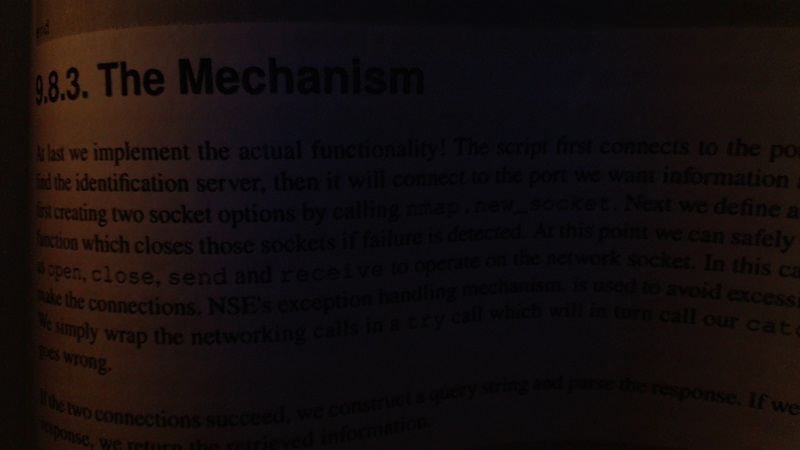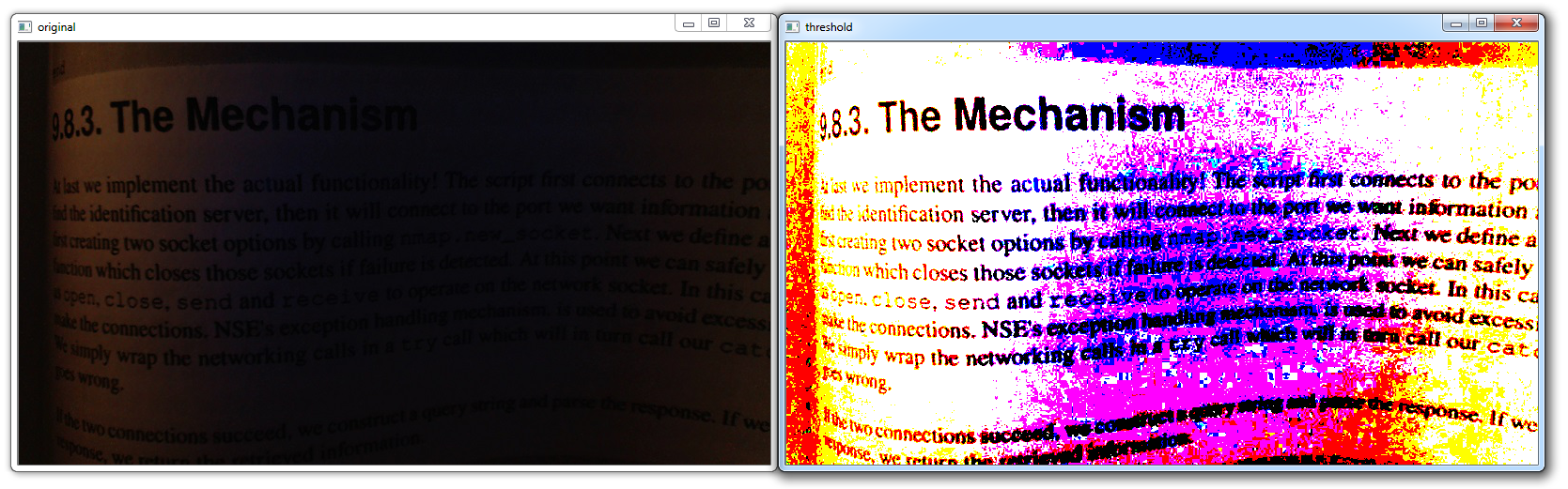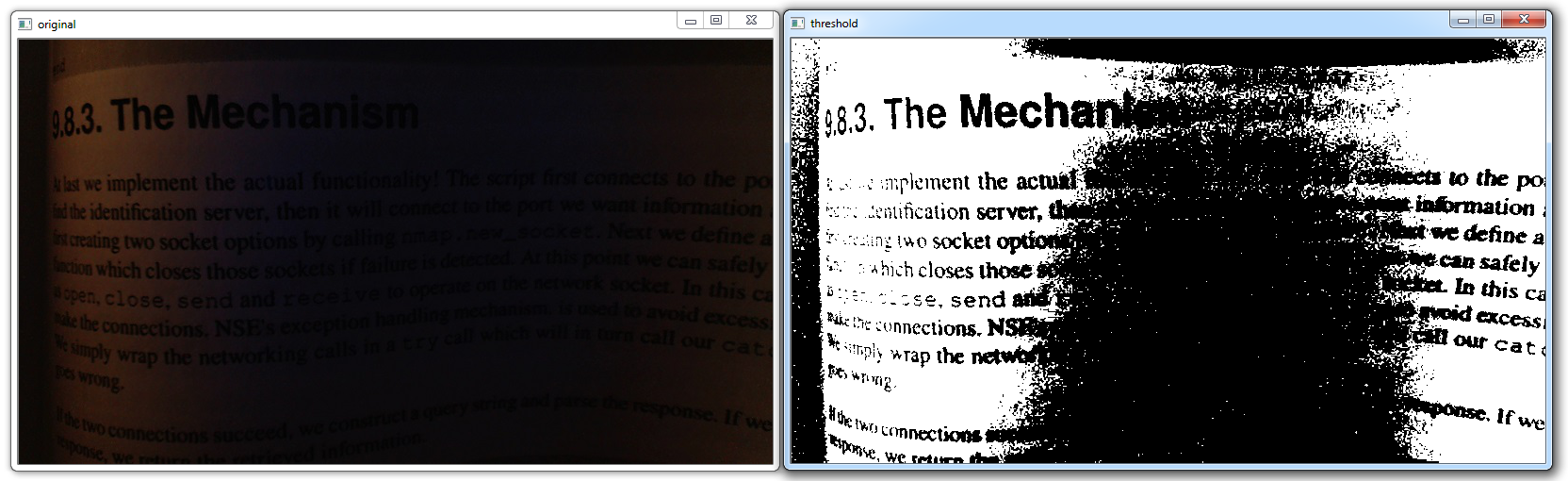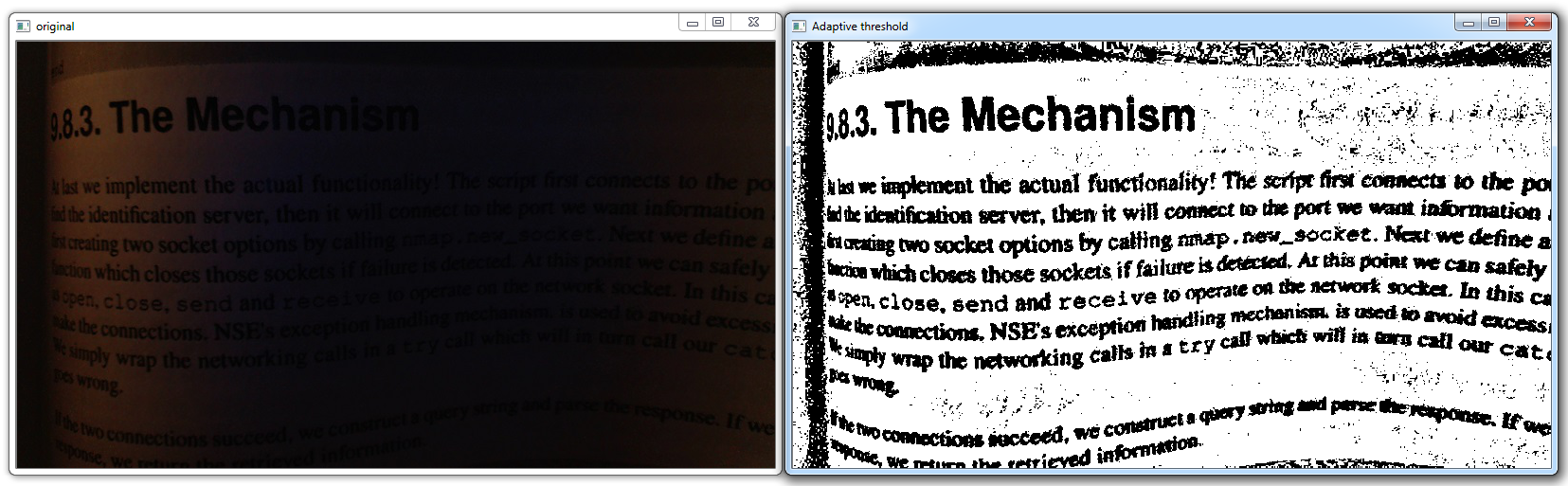## Thresholding OpenCV Python Tutorial

Welcome to another OpenCV tutorial. In this tutorial, we'll be covering thresholding for image and video analysis. The idea of thresholding is to further-simplify visual data for analysis. First, you may convert to gray-scale, but then you have to consider that grayscale still has at least 255 values. What thresholding can do, at the most basic level, is convert everything to white or black, based on a threshold value. Let's say we want the threshold to be 125 (out of 255), then everything that was 125 and under would be converted to 0, or black, and everything above 125 would be converted to 255, or white. If you convert to grayscale as you normally will, you will get white and black. If you do not convert to grayscale, you will get thresholded pictures, but there will be color.

While that sounds good enough, it often isn't. We will be covering multiple examples and different types of thresholding here to illustrate this. We will use the following image as our example image, but feel free to use one of your own:This short blurb from a book makes for a great example of why one might threshold. First, the background has really no white at all, everything is dim, but also everything is varying. Some parts are light enough to be easily read, while others are quite dark and require quite a bit of focus to make out. First, let's try just a simple threshold:

`retval, threshold = cv2.threshold(img, 10, 255, cv2.THRESH_BINARY)`

A binary threshold is a simple "either or" threshold, where the pixels are either 255 or 0. In many cases, this would be white or black, but we have left our image colored for now, so it may be colored still. The first parameter here is the image. The next parameter is the threshold, we are choosing 10. The next is the maximum value, which we're choosing as 255. Next and finally we have the type of threshold, which we've chosen as THRESH_BINARY. Normally, a threshold of 10 would be somewhat poor of a choice. We are choosing 10, because this is a low-light picture, so we choose a low number. Normally something about 125-150 would probably work best.

```import cv2
import numpy as np

retval, threshold = cv2.threshold(img, 12, 255, cv2.THRESH_BINARY)
cv2.imshow('original',img)
cv2.imshow('threshold',threshold)
cv2.waitKey(0)
cv2.destroyAllWindows()```

Result:The image now is slightly better for reading, but still a bit of a mess. Visually, it is better, but using a program to analyze this will still be quite hard. Let's see if we can simplify it further.

First, let's grayscale the image, and then do a threshold:

```import cv2
import numpy as np

grayscaled = cv2.cvtColor(img,cv2.COLOR_BGR2GRAY)
retval, threshold = cv2.threshold(grayscaled, 10, 255, cv2.THRESH_BINARY)
cv2.imshow('original',img)
cv2.imshow('threshold',threshold)
cv2.waitKey(0)
cv2.destroyAllWindows()```More simple, yep, but we're still missing out on a lot of context here. Next up, we can try adaptive thresholding, which will attempt to vary the threshold, and hopefully account for the curving pages.

```import cv2
import numpy as np

cv2.imshow('original',img)
cv2.waitKey(0)
cv2.destroyAllWindows()```There is another version of thresholding that one can do, called Otsu's threshold. It doesn't serve us well here, but:

```retval2,threshold2 = cv2.threshold(grayscaled,125,255,cv2.THRESH_BINARY+cv2.THRESH_OTSU)
cv2.imshow('original',img)
cv2.imshow('Otsu threshold',threshold2)
cv2.waitKey(0)
cv2.destroyAllWindows()```

The next tutorial:• Drawing and Writing on Image OpenCV Python Tutorial

• Image Operations OpenCV Python Tutorial

• Image arithmetics and Logic OpenCV Python Tutorial

• Thresholding OpenCV Python Tutorial
• Color Filtering OpenCV Python Tutorial

• Blurring and Smoothing OpenCV Python Tutorial

• Morphological Transformations OpenCV Python Tutorial

• Canny Edge Detection and Gradients OpenCV Python Tutorial

• Template Matching OpenCV Python Tutorial

• GrabCut Foreground Extraction OpenCV Python Tutorial

• Corner Detection OpenCV Python Tutorial

• Feature Matching (Homography) Brute Force OpenCV Python Tutorial

• MOG Background Reduction OpenCV Python Tutorial

• Haar Cascade Object Detection Face & Eye OpenCV Python Tutorial